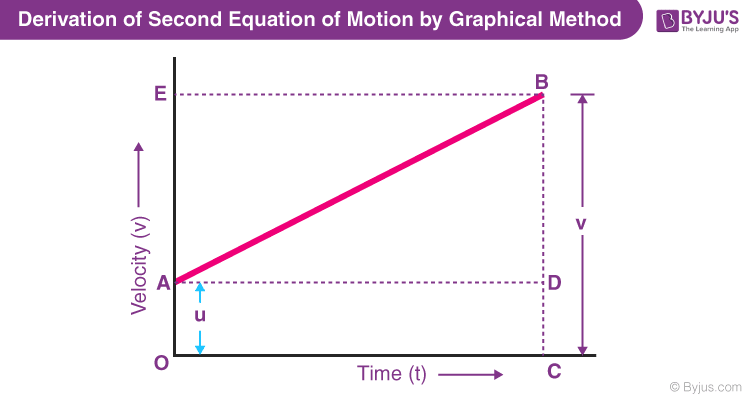# Derive the second equation of motion by graphical method

Derivation of second equation of motion by graphical method:From the graph above, we can say that

Distance travelled (s) = Area of figure OABC = Area of rectangle OADC + Area of triangle ABD

\(s=(frac{1}{2}ABtimes BD)+(OAtimes OC)\)

Since BD = EA, the above equation becomes

\(s=(frac{1}{2}ABtimes EA)+(utimes t)\)

As EA = at, the equation becomes

\(s=frac{1}{2}times attimes t+ut\)

On further simplification, the equation becomes

 \(s=ut+frac{1}{2}at^2\)